July 02, 2021

### Unpredictable Recessions

David S. Miller

This negative result indicates that recessions are not predictable in the longer-term. If the market had information regarding future recessions, that information would be reflected in the forward term spread. Whatever characteristics of today's term spread that enable it to predict near-term incoming recessions are absent in the longer-term forwards. The recession-predicting ability of term spreads enters slowly, beginning about 2 to 3 years before the recession hits. This should be reassuring: we are not doomed by fate to suffer recessions.1

For this note, I use the 10-year to 3-month Treasury term spread. This specific term spread is a common benchmark for recession prediction in the near-term, and, as shown in Miller (2019), is representative of a broad class of long-short Treasury term spreads. Monthly data on quarterly-maturity Treasury yields comes from Gurkaynak, Sack, and Wright (2006) estimates.

Define $y_t(n)$ as the yield at time $t$ of an n-quarter Treasury security. The forward rate is the implied yield on a security held between two periods in the future. At time $t$, the forward rate for an m-quarter Treasury security n-quarters ahead is

$$F_t(n,m) = \left( \frac{y_t(n+m)^{n+m}}{y_t(n)^n} \right)^{1/m}$$

In the first set of results, I construct monthly 0 to 20 quarters-ahead forwards for both 10-Year and 3-month Treasury securities starting in 1971:M11, and difference them to calculate the forward 10-Year to 3-month Treasury term spread. In the second set, I construct monthly 0 to 40 quarters-ahead forwards using data starting in 1981:M07. In both sets, I use data through 2019:M01 to avoid debate over the COVID-19 recession. Because the forward term spreads use Treasury securities of longer maturities, I also calculate the forward term spreads adjusted by the term premium estimates from Kim and Wright (2005) without surveys as described in Li, Meldrum, and Rodriguez (2017).

Recession Predicting Accuracy
To measure the power of the forward term spreads to predict recessions, I calculate a monthly probit regression for each quarter-ahead forward term spread. The independent variable is a forward term spread, and the dependent variable is an indicator that there is at least 1 NBER dated recession month within the next 12 months from the forward month. For example, in the probit using 2-year ahead forward term spreads, the 2-year ahead forward term spread in 1985:M01 corresponds to recession dates between 1987:M01 and 1988:M01.2

To measure the accuracy of the probits, I calculate the area under the receiver operator characteristic curve (AUROC). The AUROC is a statistical measure of the ratio of true positives to false positives generated by the probits. An AUROC equal to 1 indicates that all the model's predicted positives are true positives. An AUROC equal to 0.5 means half the model's predicted positives are true positives, and half are false positives; you could be equally accurate simply flipping a coin.

Figure 1 shows the AUROCs for each forward term spread probit (black line), and the same probits using forwards adjusted for the term premium (blue line), along with their respective 95% confidence intervals. To clarify how to read the figure, the AUROC of 0.7 corresponding to the 6-quarters ahead forward term spread is the measure of the accuracy of the probit that uses the 6-quarters ahead forward term premium to predict recessions that occur between 6 and 10-quarters ahead.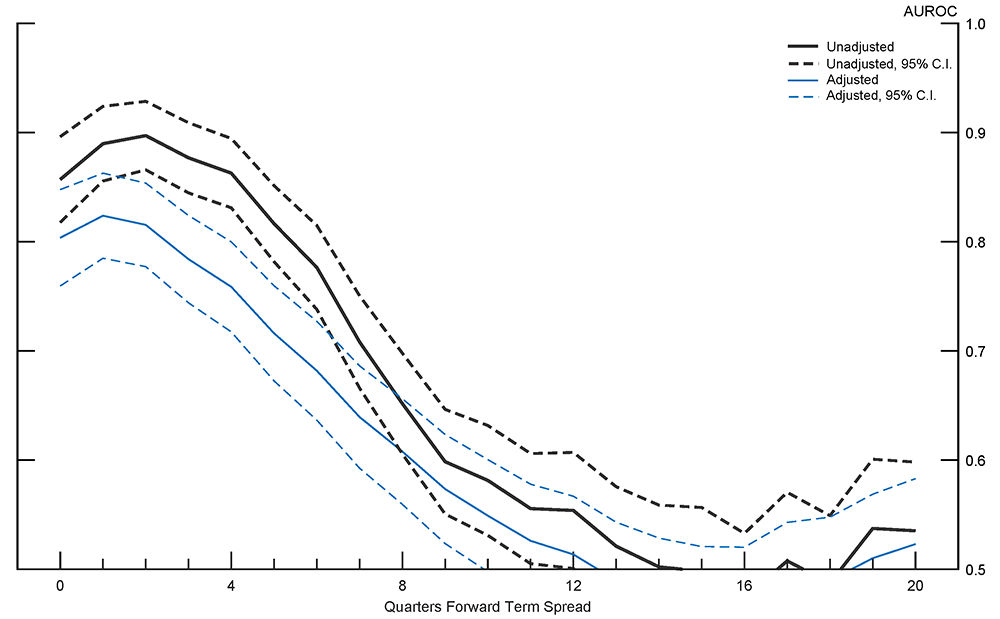Note: Full sample 1971:M11 to 2019:M01. Dashed lines indicate 95 percent confidence interval. Source: Author's calculation. Accessible version

The recession predicting accuracy of the forward term spread declines as the forward term spreads increase in years-ahead. The 2-years forward term spread, predicting a recession between 2 and 3 years from now, is just a bit better than a coin flip. Interestingly, the forwards accuracy increases over the first year, though this may be sensitive to sample selection as highlighted in Miller (2019). Adjusting for the term premium lowers the accuracy of the forward term spread, possibly because the term premium estimates are less accurate for the Treasuries with a maturity longer than 10 years that are used to calculate the forward 10-year Treasury yield, or because the term premium itself contains a measure of risk important for predicting recessions as described in Benzoni, Chyruk, and Kelley (2018).

The decline in recession predicting accuracy generally mirrors the decline in the accuracy of the forward term spreads of predicting what the term spread will be. Although the forward term spreads do not necessarily need to be accurate predictors of the term spread to be accurate predictors of recessions, obviously there could be a connection. Figure 2 plots the median absolute difference between the forward term spreads and the realized term spread for term spreads both adjusted (blue line) and unadjusted (black line) for the term premium.

##### Figure 2: Median Absolute Prediction Error of Forward Term Spreads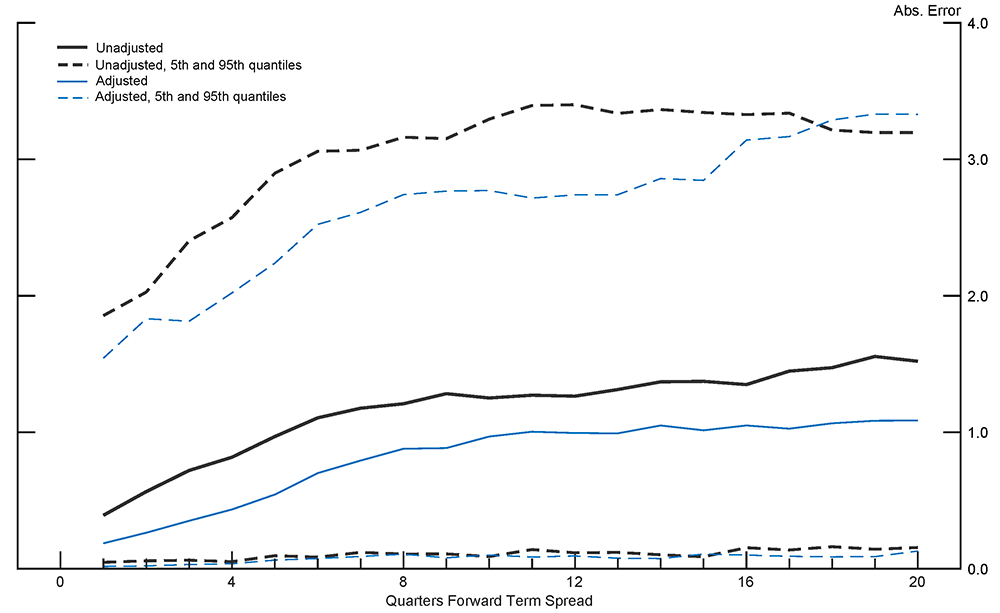Note: Full sample 1971:M11 to 2019:M01. Dashed lines indicate 5th and 95th quantiles. Source: Author's calculation. Accessible version

Interestingly, forward term spreads adjusted for the term premium are better predictors of the realized term spread than unadjusted forward term spreads, however they are slightly worse at predicting recessions. The high 95th quantiles come from around recession periods where the term spread drops sharply. The fall over the sample period of the long-term interest rate also contributes to the high level of the 95th quantile.

As a way of comparison, Figure 3 shows the AUROC for each forward term spread probit (black line), and the AUROC of a corresponding set of probits with the same NBER recession dependent variable but whose independent variable is the 10 year to 3 month Treasury term spread – not a forward – estimated over the same samples as the forward term spreads (brown line).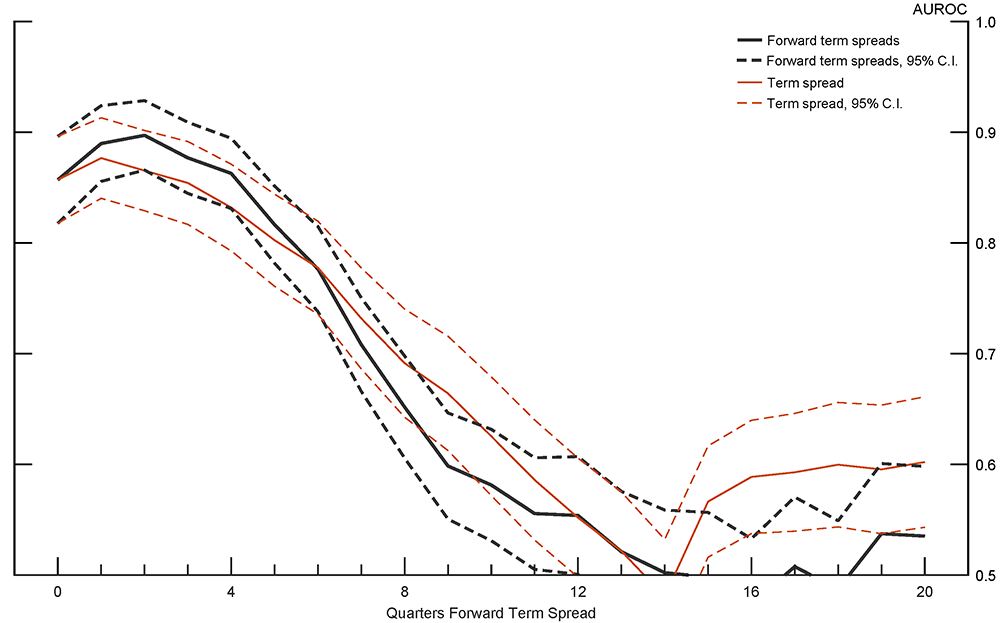Note: Full sample 1971:M11 to 2019:M01. Dashed lines indicate 95 percent confidence interval. Source: Author's calculation. Accessible version

The forwards might be a little more accurate over the first year, and the non-forward term spread a little more accurate between years 4 and 5, but the differences are minimal. The non-forward term spread appears statistically just a hair better than a coin flip between years 4 and 5. As can be seen, all the accurate recession predicting information in the yield curve is contained within the 10-year to 3-month Treasury term spread.

To test the robustness of the results, I construct the same forward term spread probits, but increase the maximum quarters-ahead from 20 to 40. The estimation sample begins in 1981:M07 and ends, as before, in 2019:M01. Overall the results are very similar to the previous analysis.

Figure 4 shows that, as before, adjusting the term spreads using term premium estimates results in minorly less accurate recession predictions. The set of AUROCs that are statistically above 0.5 centered around the 16-quarters forward term spread is not robust to sample selection as the same rise does not appear in set of results estimated from 1971. The AUROC's rise above 0.5 near the 40-quarters forward term spread also faces robustness questions as those probits are estimated on the smallest sample of data.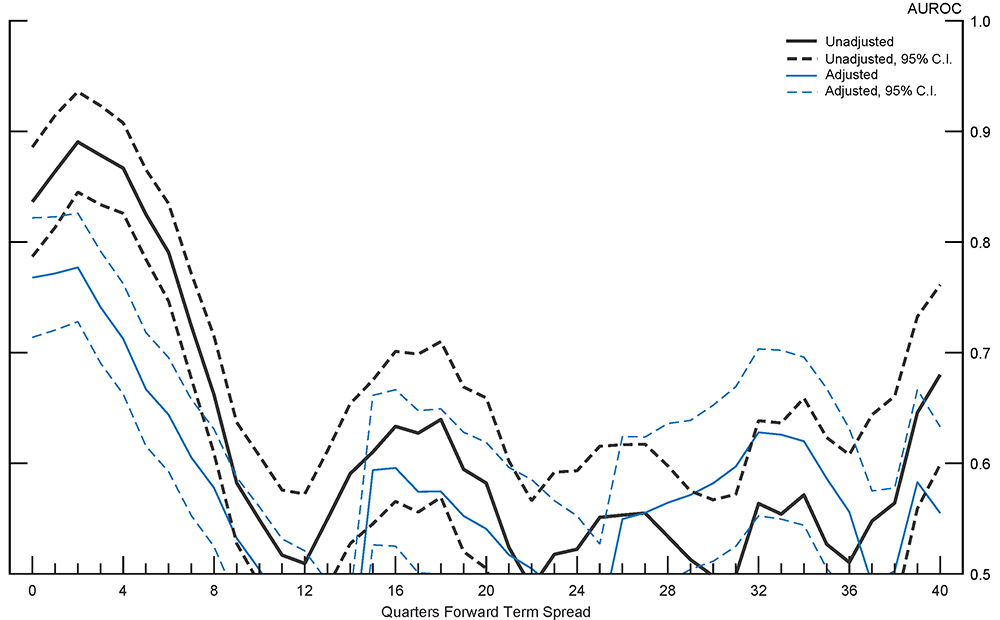Note: Full sample 1981:M07 to 2019:M01. Dashed lines indicate 95 percent confidence interval. Source: Author's calculation. Accessible version

Figure 5 illustrates that the forward term spreads are still poor predictors of what the term spread will be. The gain from adjusting for the term premium is larger than before.

##### Figure 5: Median Absolute Prediction Error of Forward Term Spreads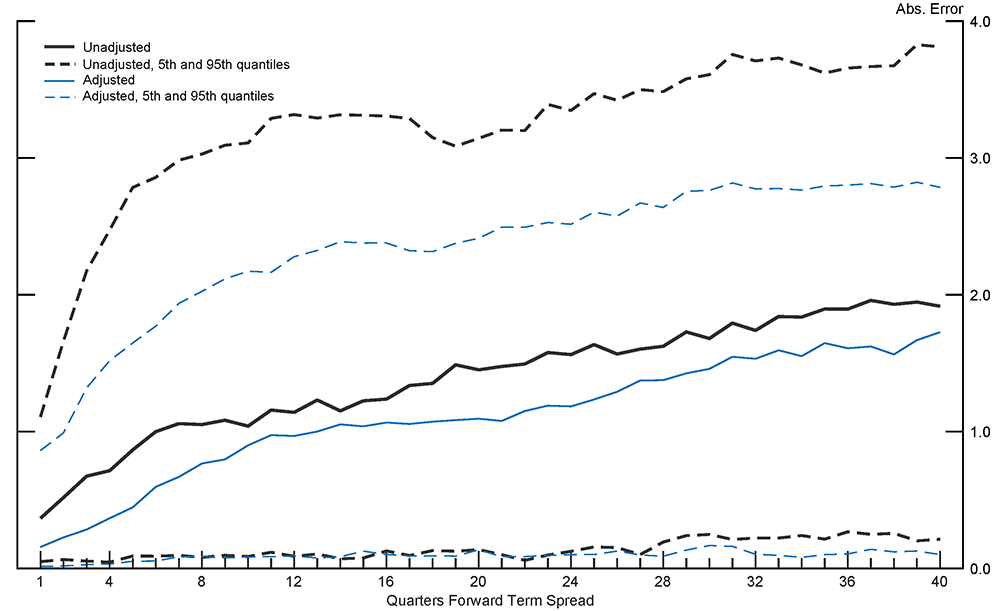Note: Full sample 1981:M07 to 2019:M01. Dashed lines indicate 5th and 95th quantiles. Source: Author's calculation. Accessible version

The forward term spreads continue to slightly underperform the accuracy of the simple 10-year to 3-month Treasury term spread as can be seen in Figure 6.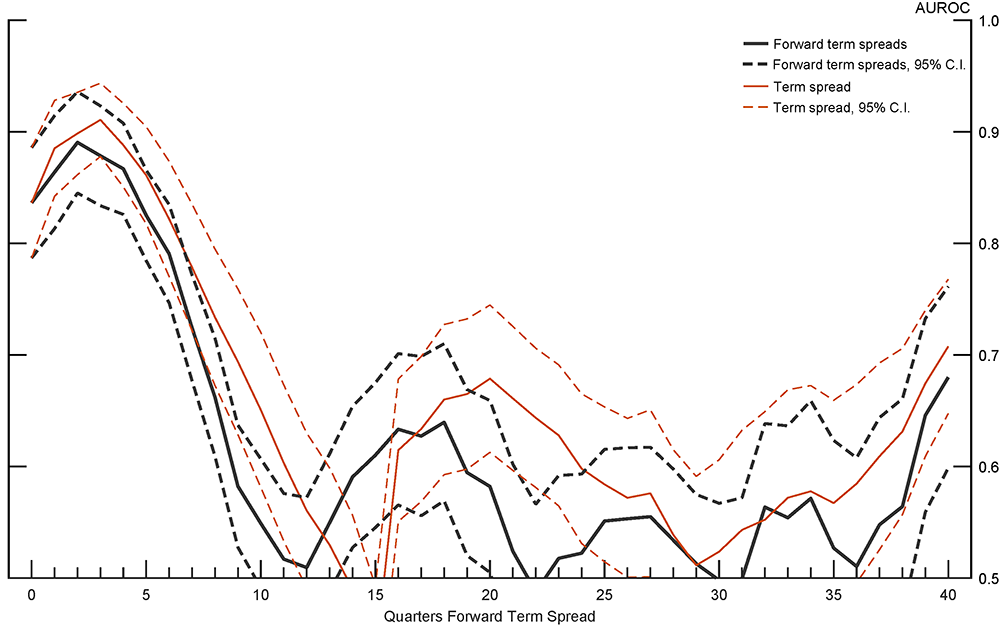Note: Full sample 1981:M07 to 2019:M01. Dashed lines indicate 95 percent confidence interval. Source: Author's calculation. Accessible version

Forward Term Spreads Recession Probability Curve
Although the AUROC analysis shows that the forward term spread probits are inaccurate at further quarters-ahead, it's interesting to take a look at what forward term spreads calculated from today's yield curve indicate about the probability of a future recession. Figure 7 presents the results of feeding forward term spreads derived from the April 2021 yield curve to the forward term spread probits to generate a probability of recession. To clarify how to read the figure, the probability of 0.2 corresponding to the 12-quarters ahead forward term spread is the probability of a recession occuring between 12 and 16-quarters ahead of April 2021.

##### Figure 7: April 2021 Forward Term Spreads Recession Probability Curve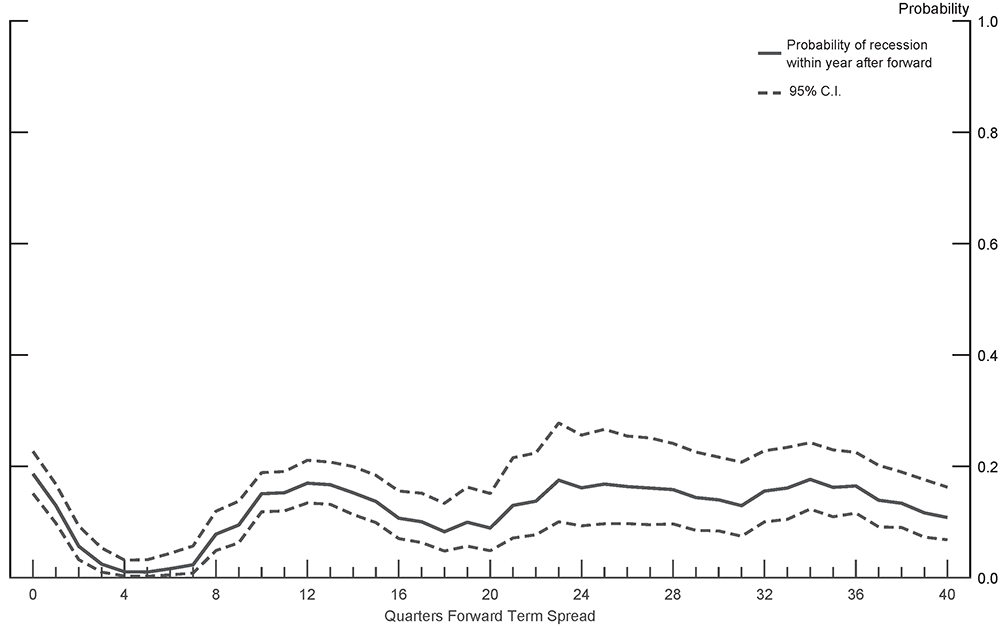Note: Probability for 2021:M04. Full sample 1981:M07 to 2019:M01. Dashed lines indicate 95 percent confidence interval. Source: Author's calculation. Accessible version

References

Benzoni, Chyruk, and Kelly, 2018. "Why Does the Yield-Curve Slope Predict Recessions", Chicago Fed Letter, Federal Reserve Bank of Chicago.

Kim and Wright, 2005, "An Arbitrage-Free Three-Factor Term Structure Model and the Recent Behavior of Long-Term Yields and Distant Horizon Forward Rates", Finance and Economics Discussion Series, 2005-33, Board of Governors of the Federal Reserve System (U.S.).

Li, Meldrum, and Rodriguez, 2017. "Robustness of Long-Maturity Term Premium Estimates," FEDS Notes 2017-04-03, Board of Governors of the Federal Reserve System (U.S.).

Miller, 2019. "There is no Single Best Predictor of Recessions," FEDS Notes, 2019-05-21, Board of Governors of the Federal Reserve System (U.S.).

1. Of course, some recessions, such as the one due to the global pandemic, are clearly both unavoidable and unpredictable. Return to text

2. This timing means that each probit is estimated on a different sample length. While the start of each sample remains the same, as the forwards increase in quarters-ahead, the sample lengths decrease because the final observation of the dependent NBER recession dating remains 2019:M01. Return to text